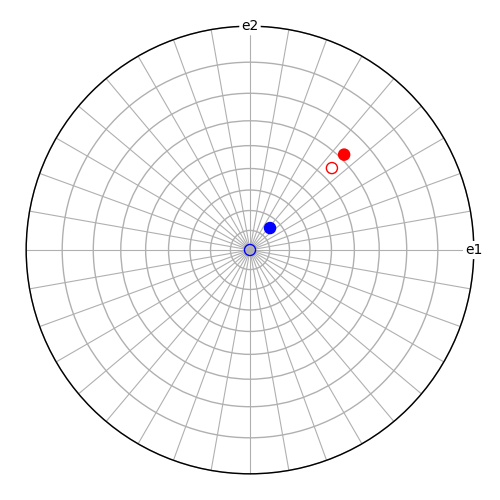# Misorientation from aligning directions#

This example demonstrates how to use `from_align_vectors()` to estimate a misorientation from two sets of aligned crystal directions, one set in each crystal reference frame.Out:

```Vector angular deviation [deg]:  1.83321960008093
Error distance:  0.004468743039965987
```

```from diffpy.structure import Lattice, Structure
import numpy as np

from orix.crystal_map import Phase
from orix.quaternion import Misorientation, Orientation
from orix.vector import Miller

# Specify two crystal structures and symmetries
phase1 = Phase(
point_group="m-3m",
structure=Structure(lattice=Lattice(1, 1, 1, 90, 90, 90)),
)
phase2 = Phase(
point_group="6/mmm",
structure=Structure(lattice=Lattice(1, 1, 2, 90, 90, 120)),
)

# Specify one orientation per crystal
o1 = Orientation.from_axes_angles(
[1, 1, 1], 60, symmetry=phase1.point_group, degrees=True
)
o2 = Orientation.from_axes_angles(
[1, 3, 2], 90, symmetry=phase2.point_group, degrees=True
)

# Get the reference misorientation (goal). Transformations are composed
# from the right, so: crystal 1 -> sample -> crystal 2
m_ref = Misorientation(o2 * (~o1), symmetry=(o1.symmetry, o2.symmetry))

# Specify two directions in the first crystal
v_c1 = Miller(uvw=[[1, 1, 1], [0, 0, 1]], phase=phase1)

# Express the same directions with respect to the second crystal
v_c2 = Miller(xyz=(m_ref * v_c1).data, phase=phase2)

# Add some randomness to the second crystal directions (0 error
# magnitude gives exact result)
error_magnitude = 0.1
v_err = Miller(xyz=np.random.normal(0, error_magnitude, 3), phase=phase2)
v_c2_err = Miller(xyz=(v_c2 + v_err).data, phase=phase2)
angle_err = v_c2_err.angle_with(v_c2, degrees=True).mean()
print("Vector angular deviation [deg]: ", angle_err)

# Get the misorientation that aligns the directions in the first crystal
# with those in the second crystal
m_new, err = Misorientation.from_align_vectors(v_c2_err, v_c1, return_rmsd=True)
print("Error distance: ", err)

# Plot the two directions in the (unrotated) first crystal's reference
# frame as open circles
fig = v_c1.scatter(
ec=["r", "b"],
s=100,
fc="none",
grid=True,
axes_labels=["e1", "e2"],
return_figure=True,
)
fig.tight_layout()

# Plot the two directions in the second crystal with respect to the
# first crystal's axes
(~m_ref * v_c2_err).scatter(c=["r", "b"], figure=fig)
```

Total running time of the script: ( 0 minutes 0.428 seconds)

Estimated memory usage: 10 MB

Gallery generated by Sphinx-Gallery